# Polygon

## What are Polygons?

• A Polygon is a closed figure made up of lines segments (not curves) in two-dimensions.
• A minimum of three line segments are required for making a closed figure, thus a polygon with a minimum of three sides is known as Triangle.

### Polygon shape

By definition, we know that the polygon is made up of line segments. Below are the shapes of some polygons that are enclosed by the different number of line segments.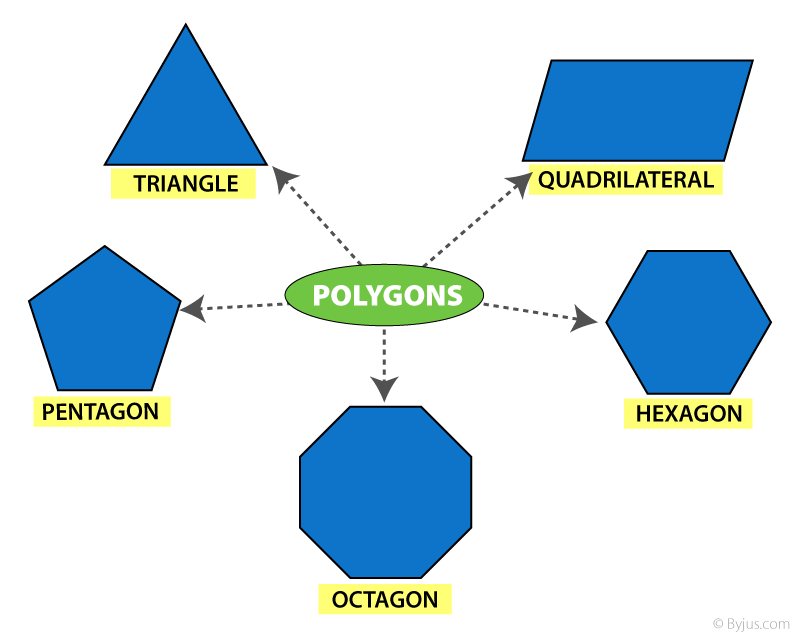## Polygon types

Depending on the sides and angles, the polygons are classified into different types, namely:

• Regular Polygon
• Irregular Polygon
• Convex Polygon
• Concave polygon

### Regular Polygon

If all the sides and interior angles of the polygon are equal, then it is known as a regular polygon.

### Irregular Polygon

If all the sides and the interior angles of the polygon are of different measure, then it is known as an irregular polygon.

### Convex Polygon

If all the interior angles of a polygon are strictly less than 180 degrees, then it is known as a convex polygon. The vertex will point outwards from the centre of the shape.

### Concave Polygon

If one or more interior angles of a polygon are more than 180 degrees, then it is known as a concave polygon. A concave polygon can have at least four sides. The vertex points towards inside of the polygon.

However, a number of polygons are defined based on the number of sides, angles and their properties. Let us see one of the frequently used and the primary type of polygon, i.e. triangle.

## Classification of Triangles

In our article on  Geometric Shapes, we discussed the polygons with the least number of sides – the Triangle. In this section, you will go through different types of triangles.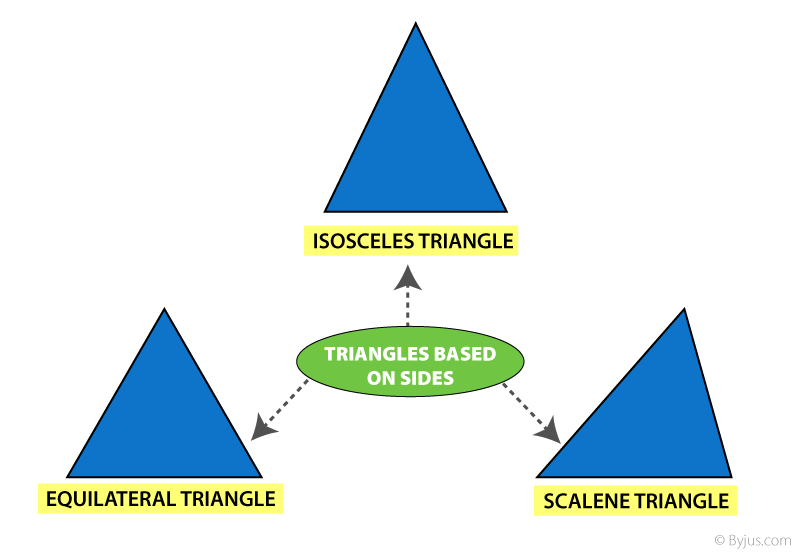• Equilateral triangle – Having all sides equal.
• Isosceles triangle – Having any 2 sides equal.
• Scalene triangle – Has 3 unequal sides.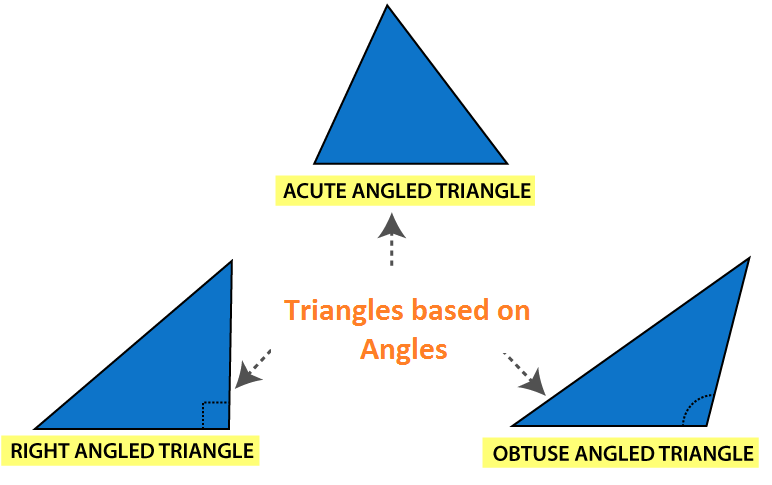• Acute angled Triangle– Each angle is less than 900
• Right Angled Triangle– Any one angle is 900
• Obtuse Angled Triangle– Any one angle is greater than 900

### An Activity:

• Draw different triangles of any dimensions using squares and measuring scales.
• Measure the length of the sides and note them down.
• You will observe that the sum of any 2 sides of the triangle is Always Greater than the 3rd side.
 An interesting fact about the Triangle: Sum of all the angles of the triangle is always equal to $180^{\circ}$ (straight angle)

A Quadrilateral is a polygon having the number of sides equal to four. That means, a polygon formed by enclosing four line segments such that they meet at each other at corners to make 4 vertices.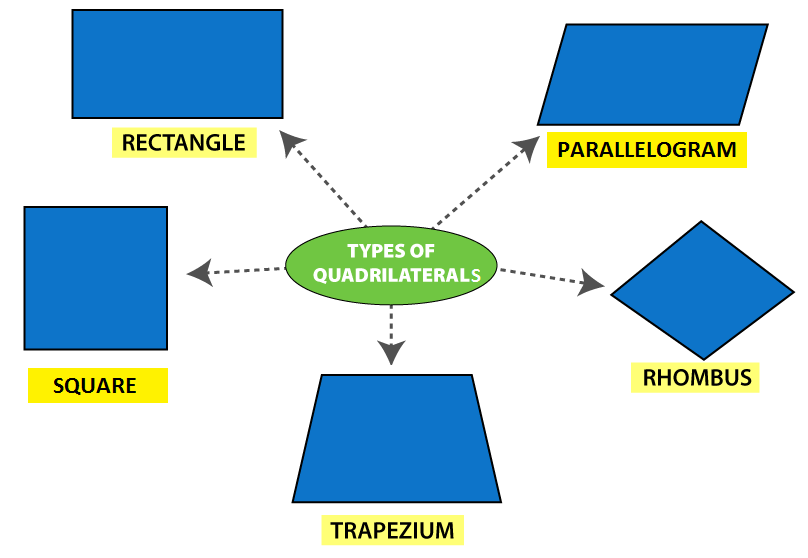The table below gives the comparison of Opposite sides, angles, and diagonals of different Quadrilateral.

 Quadrilateral Opposite Sides All sides Equal Opposite angles equal Diagonal Diagonal Parallel Equal Equal Perpendicular Rectangle ✔ ✔ ✖ ✔ ✔ ✔ Parallelogram ✔ ✔ ✖ ✔ ✖ ✖ Rhombus ✔ ✔ ✔ ✔ ✖ ✔ Trapezium ✖ (Only one side) ✖ ✖ ✖ ✖ ✖ Square ✔ ✔ ✔ ✔ ✔ ✔

### Polygon: A general comparison

 Polygon No. of Sides No. of Diagonal No. of vertices Interior Angle Triangle 3 0 3 60 Quadrilateral 4 2 4 90 Pentagon 5 5 5 108 Hexagon 6 9 6 120 Heptagon 7 14 7 128.571 Octagon 8 20 8 135 Nonagon 9 27 9 140 Decagon 10 35 10 144 Hendecagon 11 44 11 147.273 Dodecagon 12 54 12 150 Triskaidecagon 13 65 13 158.308 Tetrakaidecagon 14 77 14 154.286 Pentadecagon 15 90 15 156

### Polygon angle sum

As we know, any polygon has as many vertices as it has sides. Each corner has a certain measure of angles. These angles are categorized into two types namely interior and exterior angles of a polygon.

The sum of all the interior angles of a simple n-gon = (n − 2) × 180°
Or
Where ‘n’ is equal to the number of sides of a polygon.
For example, a quadrilateral has four sides, therefore, the sum of all the interior angle = (4 – 2) × 180°
= 2 × 180°
= 360°

The sum of interior and the corresponding exterior angles at each vertex of any polygon are supplementary to each other.

### Polygon formula

There are several formulas defined for polygons based on the number of sides.
As we have already given that the sum of all the interior angles of an n-sided polygon is (n – 2) × 180°.
The number of diagonals in a polygon with n sides = n(n – 3)/2
The number of triangles formed by joining the diagonals from one corner of a polygon = n – 2
The measure of each interior angle of n-sided regular polygon = [(n – 2) × 180°]/n
The measure of each exterior angle of an n-sided regular polygon = 360°/n

## Three Dimensional Shape (3-D shape)

A three-dimensional shape is a solid object that is formed by a combination of polygons and 2d shapes. Some of the 3d shapes which we can observe in real-life are: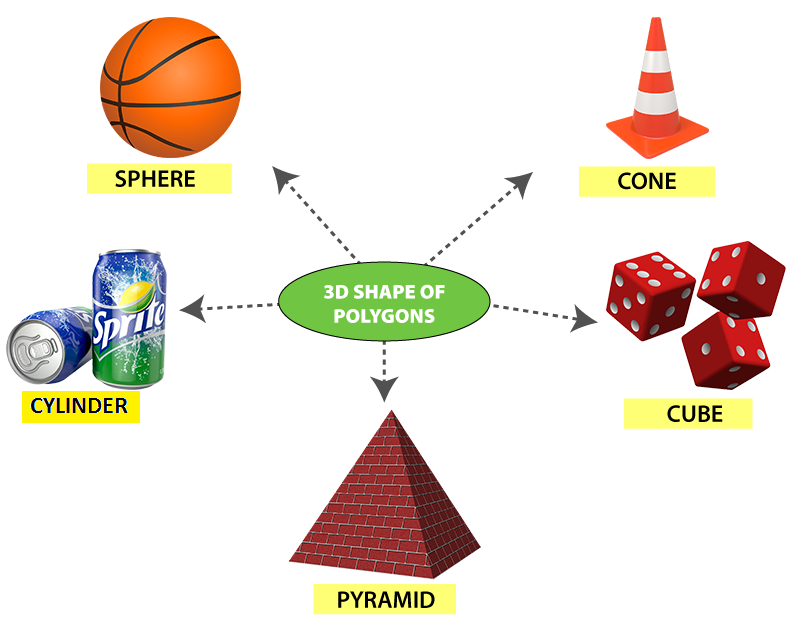• These are the shapes that can be projected on a piece of paper but cannot be drawn on a paper. These shapes are known as solids.
 2-D shape (Polygon) 3-D Shape Edge Edge Vertices Vertices — Faces
• 3-D shapes have faces as the distinguishing feature.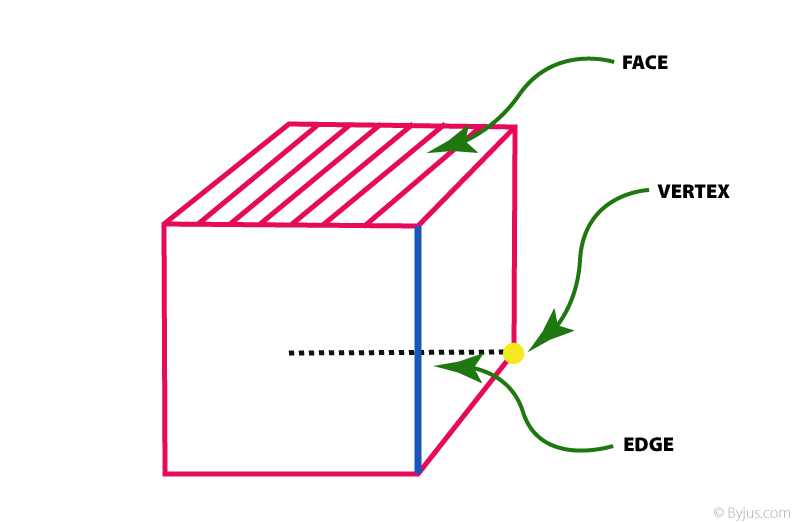To know more about polygons, types of polygons and 3-D shapes, download BYJU’S – The Learning App.

## Frequently Asked Questions – FAQs

### What is a polygon?

A polygon is a closed two-dimensional shape that is formed by enclosing line segments. A minimum of three line segments are required to make a polygon.

### What is called a polygon with 7 sides?

A polygon with 7 sides is called a Heptagon. This polygon has 7 vertices.

### What is called a polygon with 9 sides?

A polygon with 9 sides is known as Nonagon. This polygon has 9 vertices.

### How many diagonals does a polygon have?

If there are n sides in a polygon (or n-sided polygon), then the polygon will have n(n – 3)/2 diagonals. Triangles do not have diagonals.

### What are the different types of polygons?

Polygons are classified mainly into four categories. They are:
Regular polygon – all the sides and measure of interior angles are equal
Irregular polygon – all the sides and measure of interior angles are not equal, i.e. different
Convex polygon – all the interior angles of a polygon are strictly less than 180 degrees. The vertex will point outwards from the centre of the shape
Concave polygon – one or more interior angles of a polygon are more than 180 degrees. A concave polygon can have at least four sides. The vertex points towards inside of the polygon.

1. Yuvraj Tamta

This ia a great way of learning!!!!

2. Jasmine

Thank you for helping me

3. hacker

I like studing with buyjus its a very nice application

4. Ashmita

I am so happy for the conceptual understanding. It has helped me to help my students

5. Uday

This is helping to me for my studies